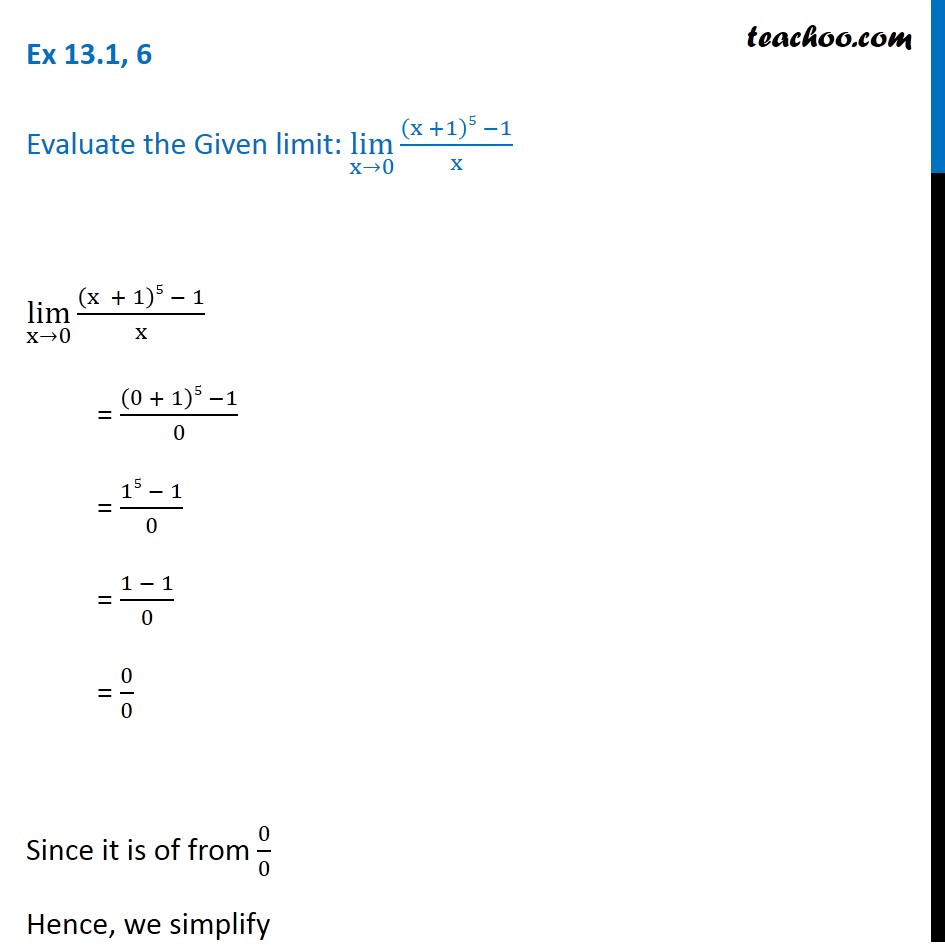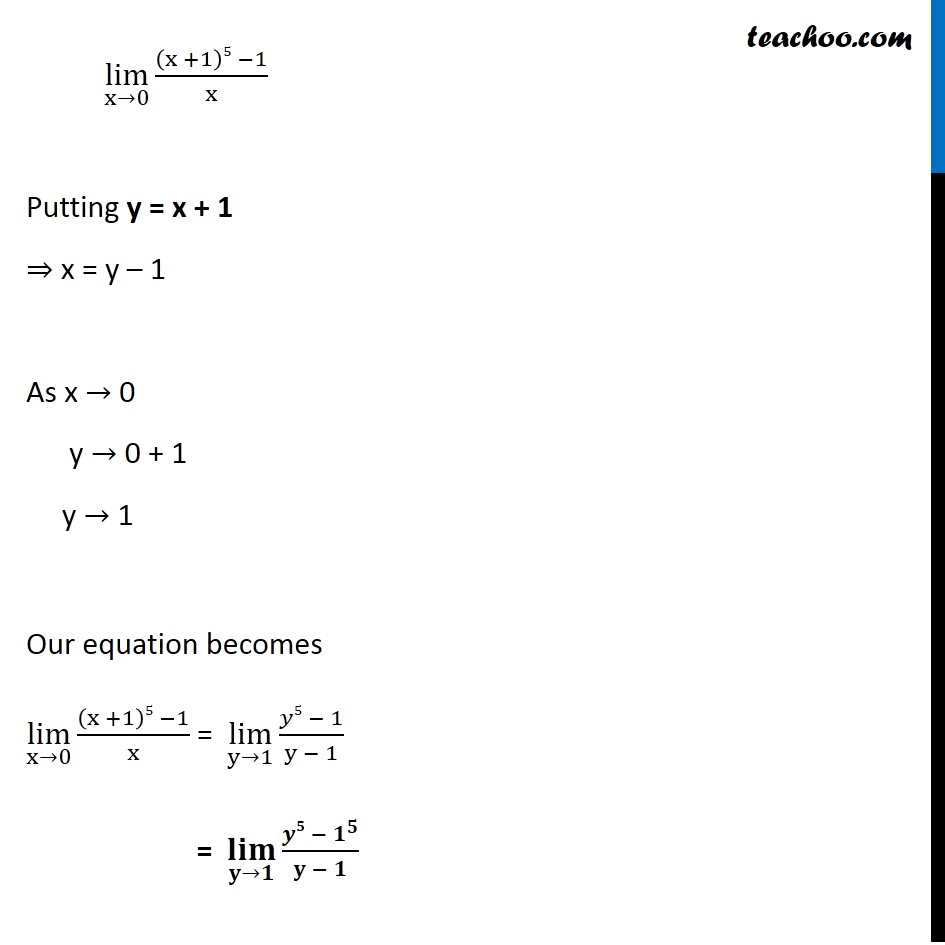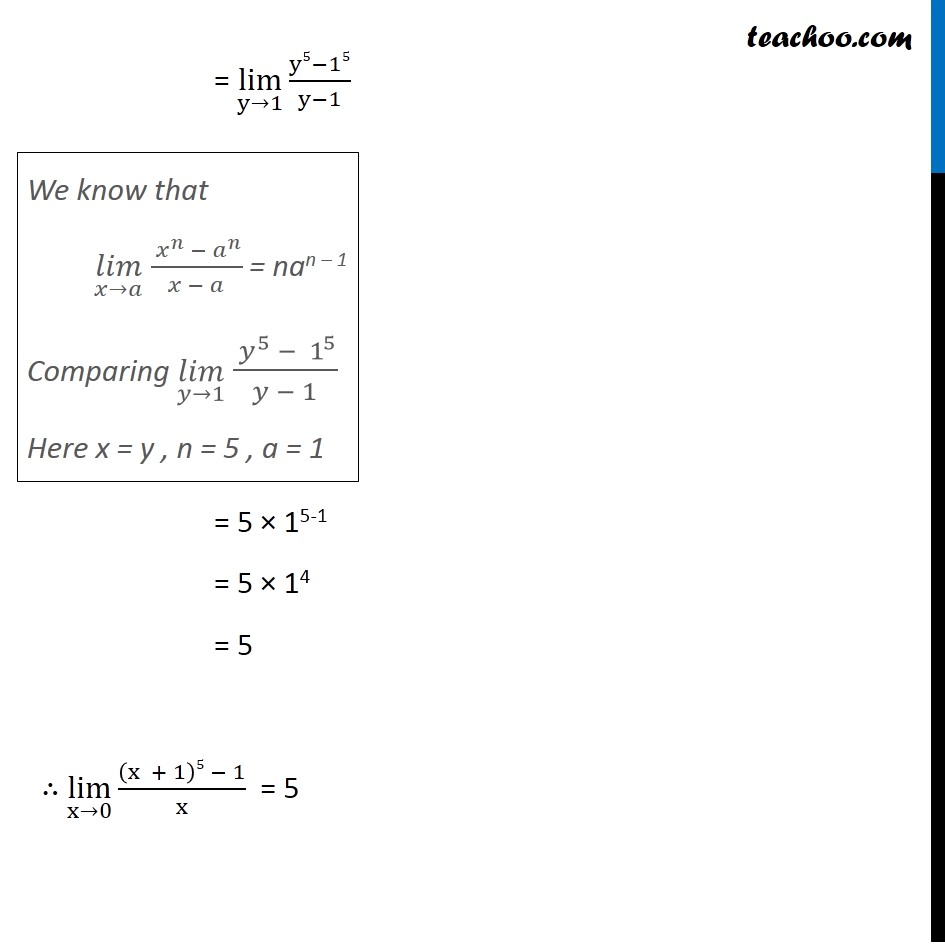1. Chapter 13 Class 11 Limits and Derivatives (Term 1 and Term 2)
2. Serial order wise
3. Ex 13.1 (Term 1)

Transcript

Ex 13.1, 6 Evaluate the Given limit: lim┬(x→0) ((x +1)5 −1)/x lim┬(x→0) ((x + 1)5 − 1)/x = ((0 + 1)5 −1)/0 = (15 − 1)/0 = (1 − 1)/0 = 0/0 Since it is of from 0/0 Hence, we simplify lim┬(x→0) ((x +1)5 −1)/x Putting y = x + 1 ⇒ x = y – 1 As x → 0 y → 0 + 1 y → 1 Our equation becomes lim┬(x→0) ((x +1)5 −1)/x = lim┬(y→1) (𝑦5 − 1)/(y − 1) = (𝐥𝐢𝐦)┬(𝐲→𝟏) (𝒚𝟓 − 𝟏^𝟓)/(𝐲 − 𝟏) = lim┬(y→1) (y5−15)/(y−1) = 5 × 15-1 = 5 × 14 = 5 ∴ lim┬(x→0) ((x + 1)5 − 1)/x = 5 We know that (𝑙𝑖𝑚)┬(𝑥→𝑎) ( 𝑥^𝑛 − 𝑎^𝑛)/(𝑥 − 𝑎) = nan – 1 Comparing (𝑙𝑖𝑚)┬(𝑦→1) ( 𝑦^5 − 1^5)/(𝑦 − 1) Here x = y , n = 5 , a = 1

Ex 13.1 (Term 1)